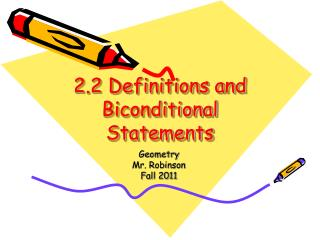DownloadDownload Presentation2.2 Definitions and Biconditional Statements

# 2.2 Definitions and Biconditional Statements

Download Presentation## 2.2 Definitions and Biconditional Statements

- - - - - - - - - - - - - - - - - - - - - - - - - - - E N D - - - - - - - - - - - - - - - - - - - - - - - - - - -
##### Presentation Transcript

1. 2.2 Definitions and Biconditional Statements Geometry Mr. Robinson Fall 2011

2. Essential Question: What is a Biconditional and how do I use it?

3. Definitions • Definitions: perpendicular lines are those which intersect to form a right angle • A line perpendicular to a plane is a line that intersects the plane at a point and is perpendicular to every line in the plane that intersects it.

4. Recognizing and using definitions • All definitions can be interpreted “forward” and “backward.” • The definition of perpendicular lines means • If two lines are perpendicular, then they intersect to form a right angle, and • If two lines intersect to to form a right angle, then they are perpendicular.

5. Example 1 • Decide whether each statement about the diagram is true. Explain your answers. • Points D, X, and B are collinear. • AC is perpendicular to DB. • Angle AXB is adjacent to and CXD. X

6. Example 1 • Decide whether each statement about the diagram is true. Explain your answers. • True. Three points are collinear if they lie on the same point. • True. The right angle symbol in the diagram indicates that lines AC and DB intersect to form a right angle; so the lines are perpendicular. • False. By definition, adjacent angles must share a common side. They do not, so are not adjacent. X

7. Using Biconditional Statements • A biconditional statement is one that contains the phrase “if and only if.” Writing a biconditional statement is equivalent to writing a conditional statement and its converse.

8. Example 2 • The biconditional statement below can be rewritten as a conditional statement and its converse. • Three lines are coplanar if and only if they lie in the same plane. • Conditional statement: If three lines are coplanar, then they lie in the same plane. • Converse: If three lines lie in the same plane, then they are coplanar.

9. Biconditional Statements • A biconditional statement can either be true or false. To be true, BOTH the conditional statement and its converse must be true. This means that a true biconditional statement is true both “forward” and “backward.” All definitions can be written as true biconditional statements.

10. Example 3: Analyzing Biconditional Statements • Consider the following statement: x = 3 if and only if x2 = 9. • Is this a biconditional statement? • The statement is biconditional because it contains the phrase “if and only if.” • Is the statement true? • Conditional statement: If x = 3, then x2 = 9. • Converse: x2 = 9, then x = 3. • The first part of the statement is true, but what about -3? That makes the second part of the statement false.

11. Example 4: Writing a Biconditional Statement • Each of the following is true. Write the converse of each statement and decide whether the converse is true or false. If the converse is TRUE, then combine it with the original statement to form a true biconditional statement. If the statement is FALSE, then state a counterexample. • If two points lie in a plane, then the line containing them lies in the plane.

12. Example 4: Writing a Biconditional Statement • Converse: If a line containing two points lies in a plane, then the points lie in the plane. The converse is true as shown in the diagram on page 81. It can be combined with the original statement to form a true biconditional statement written below: • Biconditional statement: Two points lie in a plane if and only if the line containing them lies in the plane.

13. Example 4: Writing a Biconditional Statement • B. If a number ends in 0, then the number is divisible by 5. • Converse: If a number is divisible by 5; then the number ends in 0. • The converse isn’t true. What about 25? • Knowing how to use true biconditional statements is an important tool for reasoning in Geometry. For instance, if you can write a true biconditional statement, then you can use the conditional statement or the converse to justify an argument.

14. Example 4: Writing a Biconditional Statement • B. If a number ends in 0, then the number is divisible by 5. • Converse: If a number is divisible by 5; then the number ends in 0. • The converse isn’t true. What about 25? • Knowing how to use true biconditional statements is an important tool for reasoning in Geometry. For instance, if you can write a true biconditional statement, then you can use the conditional statement or the converse to justify an argument.

15. Example 5: Writing a Postulate as a Biconditional Statement • The second part of the Segment Addition Postulate is the converse of the first part. Combine the statements to form a true biconditional statement. • If B lies between points A and C, then AB + BC = AC. • Converse: If AB + BC = AC; then B lies between points A and C. • Combine these statements

16. Example 5: Writing a Postulate as a Biconditional Statement • Point B lies between points A and C if and only if AB + BC = AC.

17. Assignment: • pp. 82-83 #2-42 even